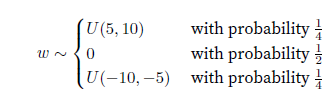# Parameter Management

Chap 5.2.2.1
in the first and second pytorch code, for function
init_normal(m)
I guess it should be
nn.init.XXX(m.weight, *args)
since

pytorch.nn.Module.apply(fn) Applies `fn` recursively to every submodule (as returned by `.children()` ) as well as self.

it doesn’t make sense to repeatedly initialize net and it’s aiming to initialize all parameters

Hi @kwang that’s a great catch. If we are to apply initialization to all the `Linear layers` in the network, then we should replace `net` to `m` inside the `init_normal` function.

Ps. While I was at it, I have fixed the naming of the functions too. The second function should be named `init_constant`.

This is now fixed in master. You can soon see the changes in the next update to release branch.
Thanks!

For tied parameters (link), why is the gradient the sum of the gradients of the two layers? I was thinking it would be the product of the gradients of the two layers. Reasoning:

y = f(f(x))
dy/dx = f’(f(x))*f’(x) where x is a vector denoting the shared parameters.

1 Like

Maybe the grad of the shared layer is not reset to 0 after the first time we meet it, so the grad of the shared layer would be computed twice and the final grad would be the sum?

For tied parameters 5.2.3 `print(net.weight.data == net.weight.data)`, I am guessing it should have been `print(net.weight.data == net.weight.data)`, given parameters are shared among layer 2 and layer 3.

Layer 2 and Layer 3 have a ReLU between them
therefore

``````net
``````

would index the ReLU
that is why its

I have a doubt in the “Custom Initialization section”.``````def my_init(m):
if type(m) == nn.Linear:
print(
"Init",
*[(name, param.shape) for name, param in m.named_parameters()])
nn.init.uniform_(m.weight, -10, 10)
m.weight.data *= m.weight.data.abs() >= 5
net.apply(my_init)
net.weight[:2]
``````

where is the probability thing implemented in thecode given in the book?

If you see it closely, this is already incorporated. The values between -5 and 5 have a probability of 0.5 to occur and those are being converted to 0.

m.weight.data.abs() >= 5

This return True(or 1) if it is true and False(0) if 0 if it is false and it is being taken care here.